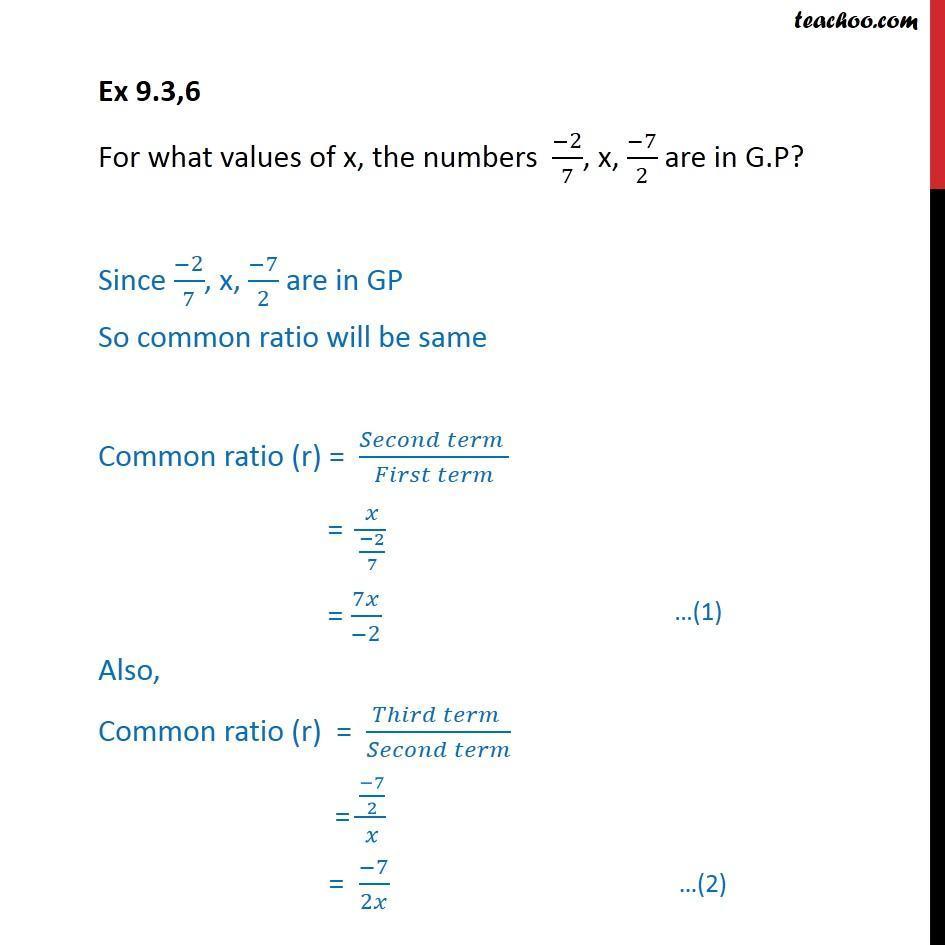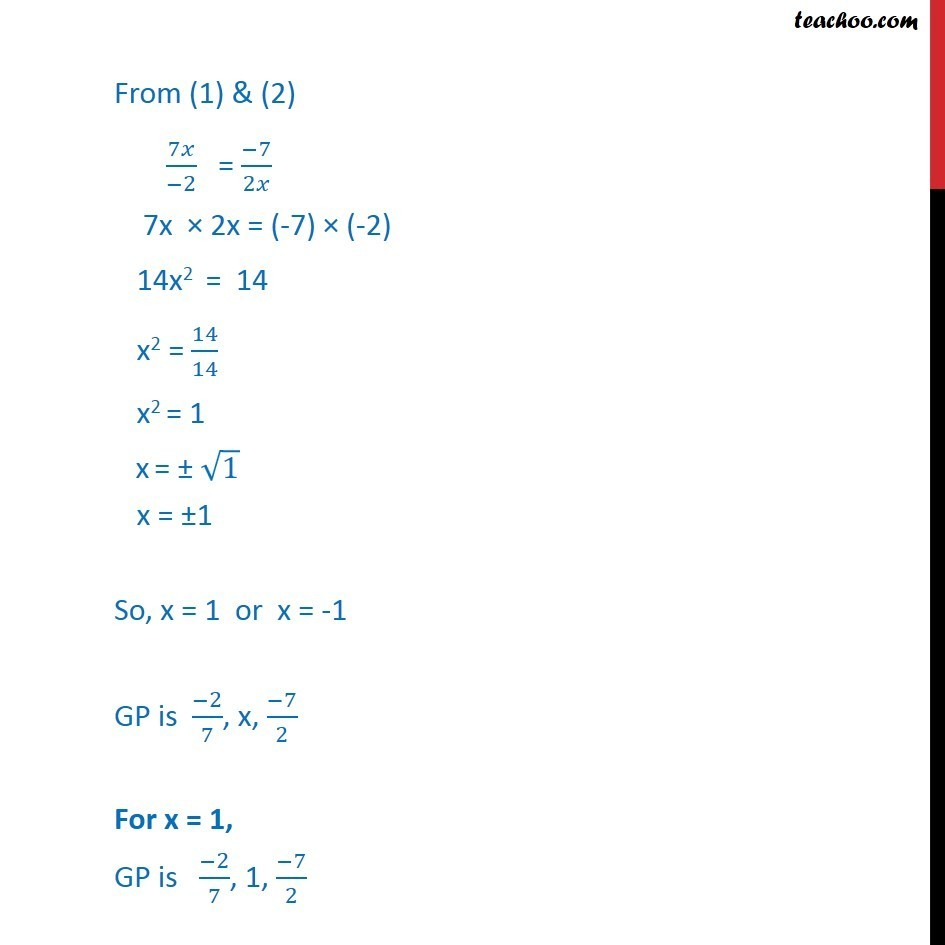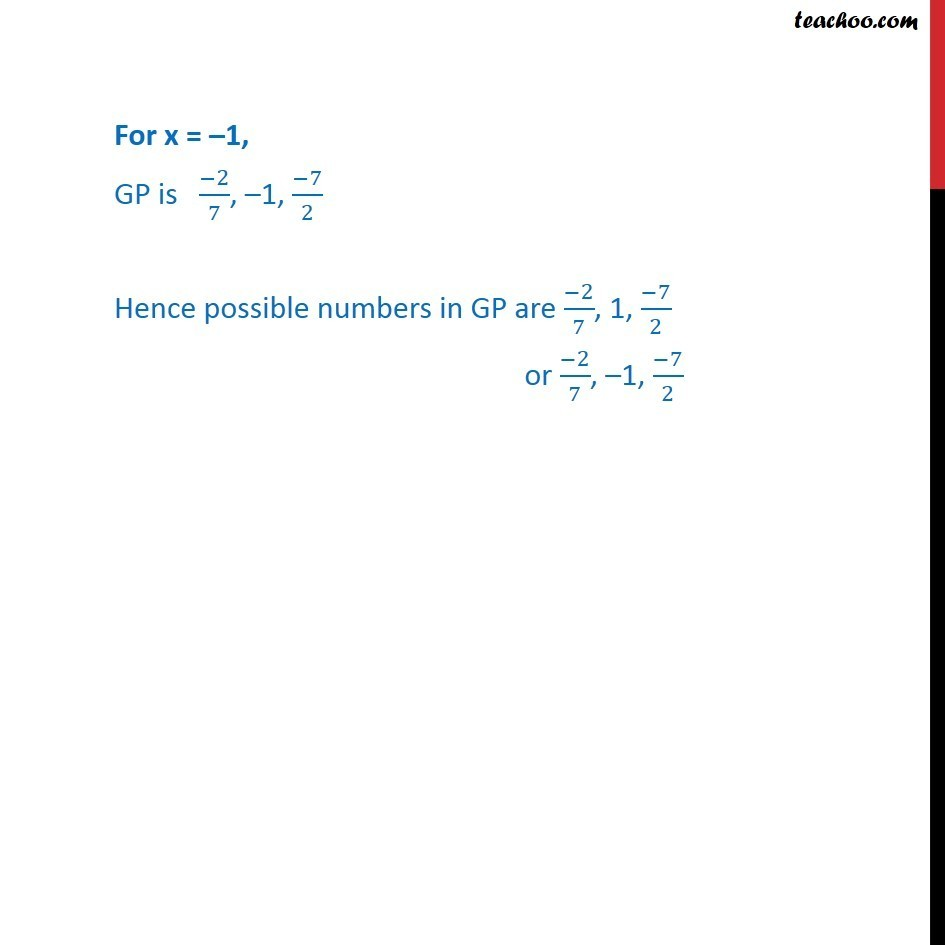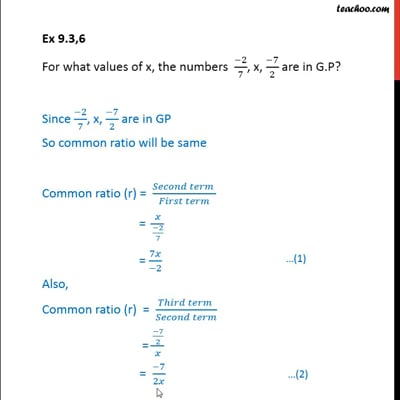Ex 9.3

Chapter 9 Class 11 Sequences and Series
Serial order wiseThis video is only available for Teachoo black users

Introducing your new favourite teacher - Teachoo Black, at only ₹83 per month

### Transcript

Ex 9.3,6 For what values of x, the numbers (−2)/7, x, (−7)/2 are in G.P? Since (−2)/7, x, (−7)/2 are in GP So common ratio will be same Common ratio (r) = (𝑆𝑒𝑐𝑜𝑛𝑑 𝑡𝑒𝑟𝑚 )/(𝐹𝑖𝑟𝑠𝑡 𝑡𝑒𝑟𝑚) = 𝑥/((−2)/7) = 7𝑥/(−2) Also, Common ratio (r) = (𝑇ℎ𝑖𝑟𝑑 𝑡𝑒𝑟𝑚 )/(𝑆𝑒𝑐𝑜𝑛𝑑 𝑡𝑒𝑟𝑚) = ((−7)/2)/𝑥 = (−7)/2𝑥 From (1) & (2) 7𝑥/(−2) = (−7)/2𝑥 7x × 2x = (-7) × (-2) 14x2 = 14 x2 = 14/14 x2 = 1 x = ± √1 x = ±1 So, x = 1 or x = -1 GP is (−2)/7, x, (−7)/2 For x = 1, GP is (−2)/7, 1, (−7)/2 For x = –1, GP is (−2)/7, –1, (−7)/2 Hence possible numbers in GP are (−2)/7, 1, (−7)/2 or (−2)/7, –1, (−7)/2